Greater than, less than in decimals

Chapter 2 Class 7 Fractions and Decimals
Concept wise

Let’s learn this by examples

Compare 42.875 &  423.95

Comparing digits before decimal points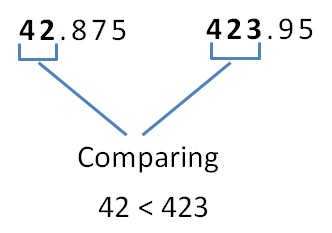So, 42.875 < 423.95

First of all, we compare the numbers before decimal points.

If they are less than or greater than,

Then the numbers are also less than or greater than

But….

What if the numbers before Decimal points are equal?

### Compare 23.75 & 23.89

Comparing digits before decimal point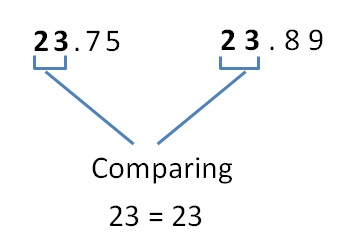So, we compare digits after decimal point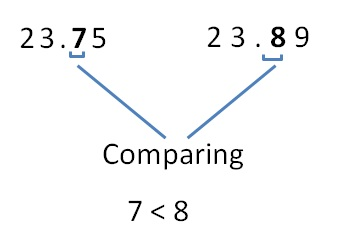So,

23.75 < 23.89

Let’s take another example

#### Compare 4.08 & 4.076

Here, number of digits of 4.08 and 4.076 is not same

Writing

4.08 = 4.080

So, we need to compare

4.080 & 4.076

Comparing digits before decimal point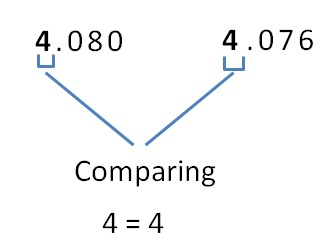So, we compare digits after decimal point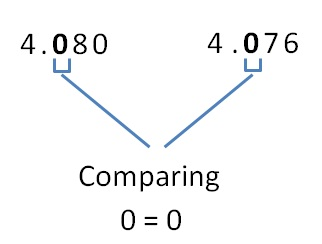So we compare the next digit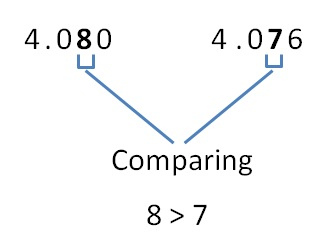So,

4.08 > 4.076

641 students have Teachoo Black. What are you waiting for?

### Transcript

Compare 23.75 & 23.89 Comparing digits before decimal point 23 = 23 So, we compare digits after decimal point 7 < 8